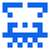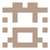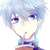# 3305. 十亿分考from fractions import Fraction
s = Fraction(float(input())).limit_denominator(int(1e9))
print(s.numerator, s.denominator)Fraction模块很好用 除了这个 OJ常用黑科技python模块还有re numpy等等